Question

# Using aggregate demand (AD), short-run aggregate supply (SRAS) and long-run aggregate supply (LRAS) curves

Using aggregate demand (AD), short-run aggregate supply (SRAS) and long-run aggregate supply (LRAS) curves, graphically illustrate the effect of an increase in the money supply on output and prices in the short and long run. Assume that the economy is initially in long run equilibrium at the potential output level and prices are fixed in the short-run. In your graph, label "A" for the initial equilibrium, "B' for the short-run equilibrium, and "C" for the long-run equilibrium.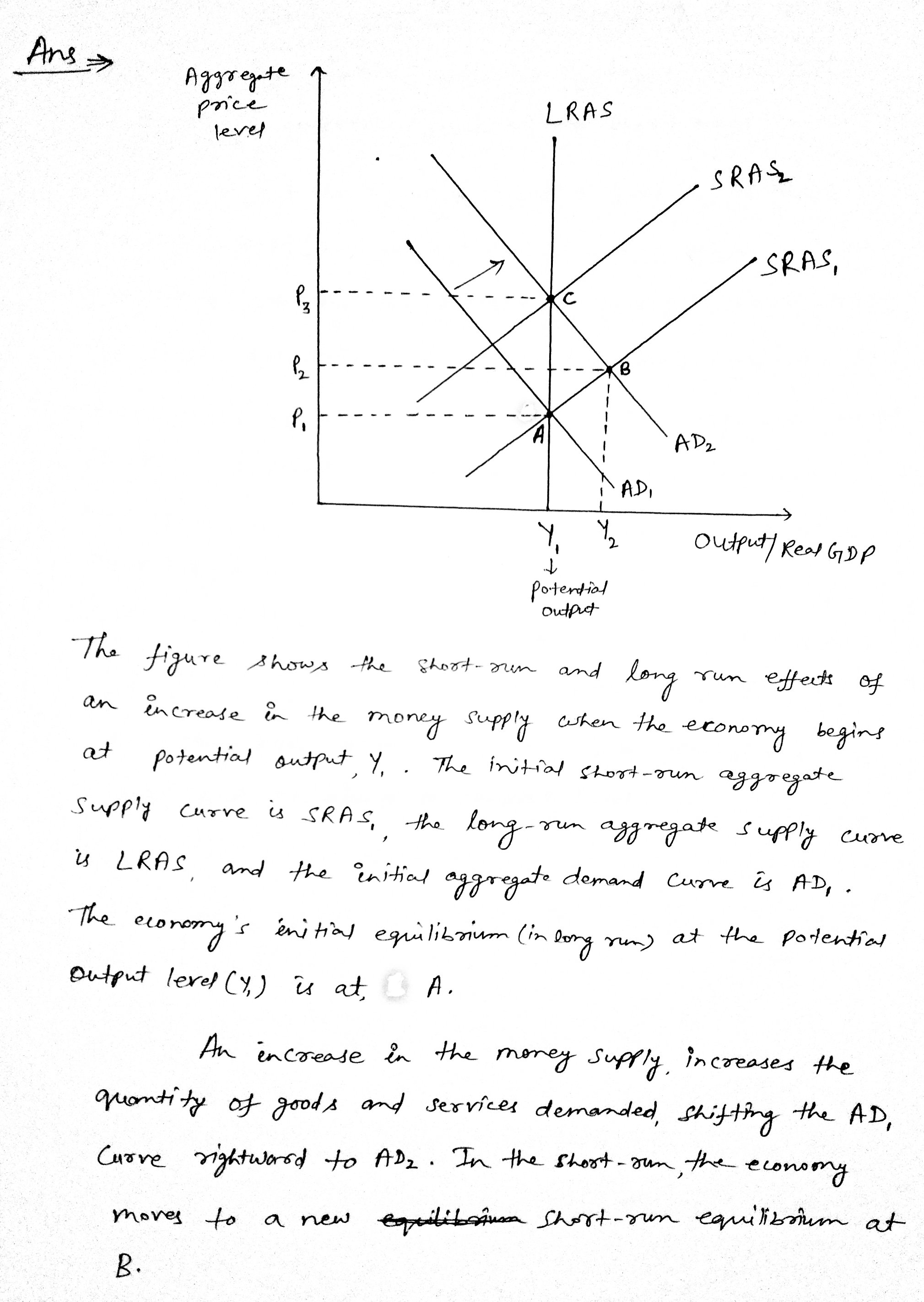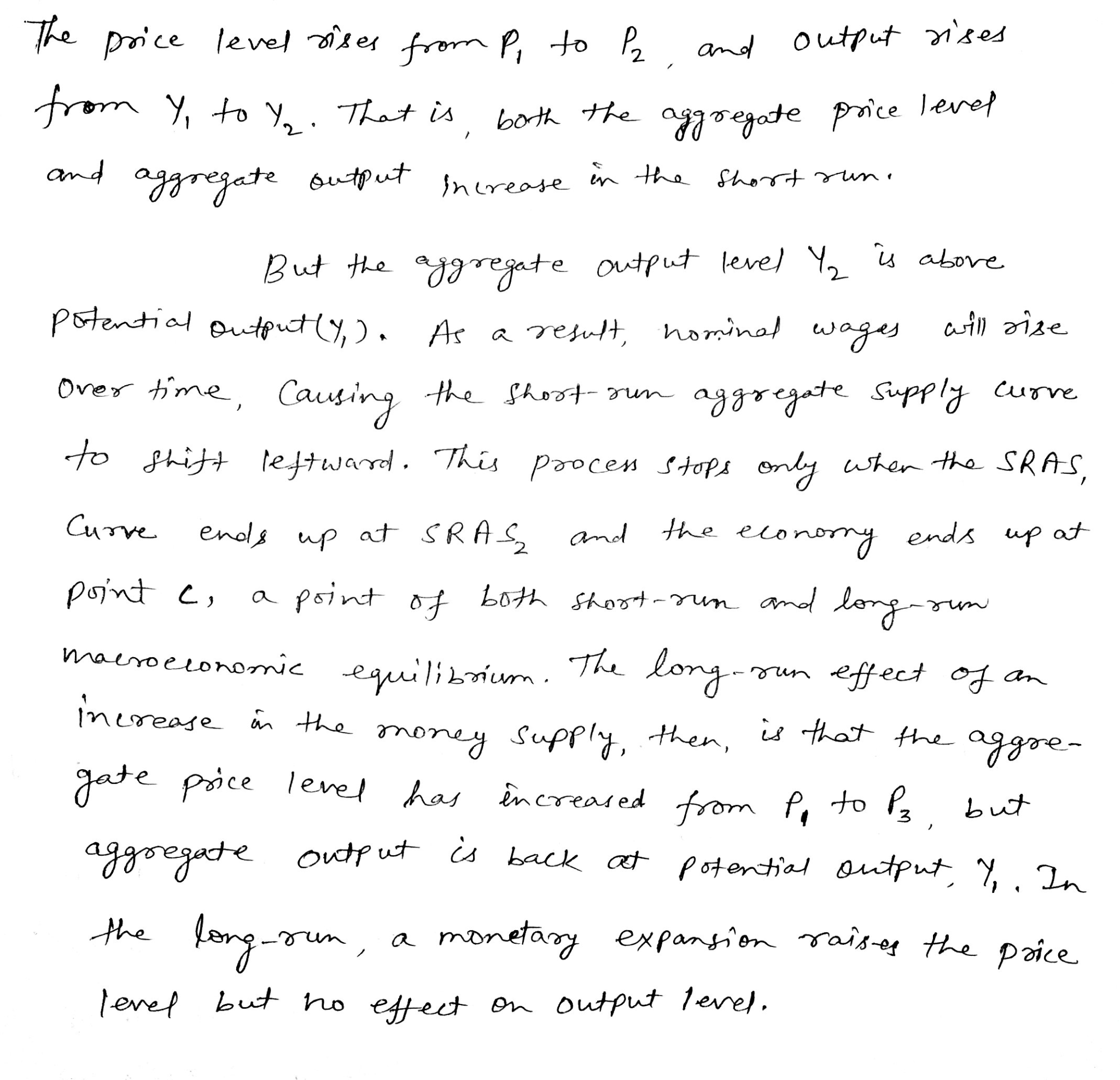#### Earn Coins

Coins can be redeemed for fabulous gifts.

Similar Homework Help Questions
• ### The following figure depicts the aggregate demand (AD), theshort-run aggregate supply (SRAS), and the long-run...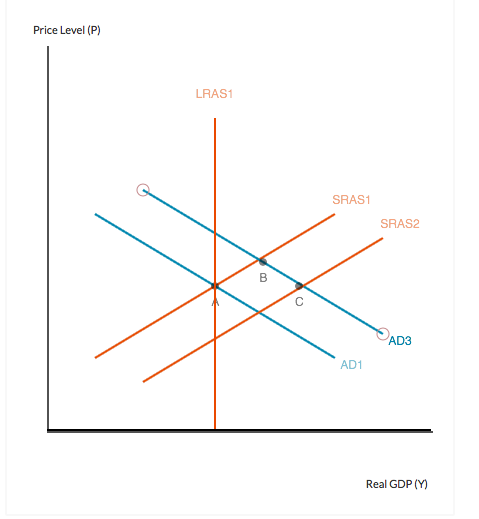The following figure depicts the aggregate demand (AD), the short-run aggregate supply (SRAS), and the long-run aggregate supply (LRAS) curves for an economy. The economy is initially at long-run equilibrium, at point A. Suppose that there is an increase in the amount of investment in the economy due to a reduction in the real interest rate. This increase in investment shifts the AD curve to the right, depicted below in the movement of the economy from point A to point...

• ### QUESTION 7 (25 points): Economic Fluctuation using AD-AS framework Suppose that the short-run aggregate supply curve...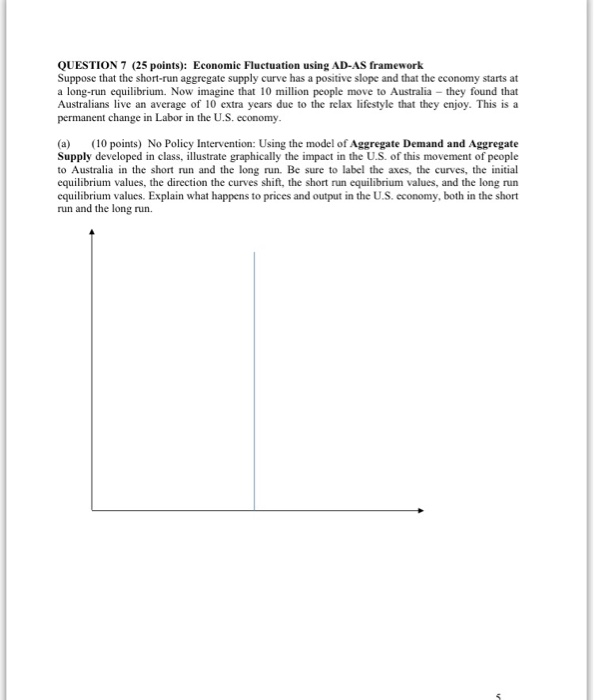QUESTION 7 (25 points): Economic Fluctuation using AD-AS framework Suppose that the short-run aggregate supply curve has a positive slope and that the economy starts at a long-run equilibrium. Now imagine that 10 million people move to Australia they found that Australians live an average of 10 extra years due to the relax lifestyle that they enjoy. This is a permanent change in Labor in the U.S. economy. (a) (10 points) No Policy Intervention: Using the model of Aggregate Demand...

• ### Describe the​ short-run aggregate supply ​(SRAS​) curve and the​ long-run aggregate supply ​(LRAS​) curve. A. the...

Describe the​ short-run aggregate supply ​(SRAS​) curve and the​ long-run aggregate supply ​(LRAS​) curve. A. the SRAS curve is horizontal and the LRAS curve is upward sloping B. the SRAS curve is horizontal and the LRAS curve is vertical C. the SRAS curve is vertical and the LRAS curve is horizontal D. the SRAS curve is vertical and the LRAS curve is upward sloping Why is the​ short-run aggregate supply curve​ horizontal? A. because output is fixed in the short...

• ### The graph below depicts the aggregate demand, Irrun aggregate supply, and short-run aggregate supply curves for...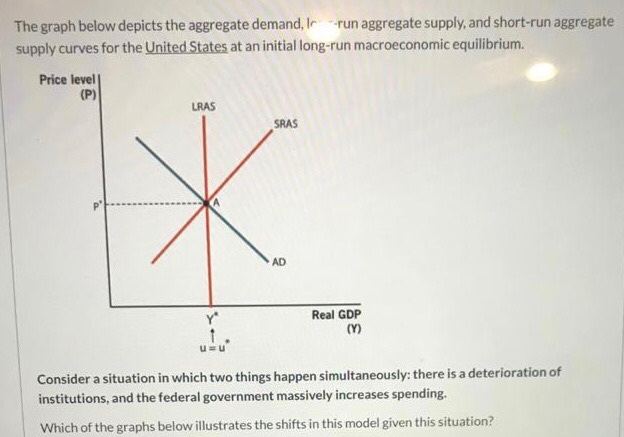The graph below depicts the aggregate demand, Irrun aggregate supply, and short-run aggregate supply curves for the United States at an initial long-run macroeconomic equilibrium Price level] (P) LRAS SRAS Real GDP Consider a situation in which two things happen simultaneously: there is a deterioration of institutions, and the federal government massively increases spending. Which of the graphs below illustrates the shifts in this model given this situation? Price level Price level (P) (P) URAS LRAS, LRAS SRAS SRAS SRAS...

• ### a) Provide a factor that would shift the long-run aggregate supply (LRAS) curve to the right....

a) Provide a factor that would shift the long-run aggregate supply (LRAS) curve to the right. What does this shift in LRAS imply for aggregate output? Use the Aggregate Demand and Supply model to illustrate this event. Make sure you properly label all the axes and curves. (You only need to draw a shift in LRAS curve, no need to draw other curves). b) Provide a factor that would shift the short-run aggregate supply (SRAS) curve upward (and to the...

• ### The following graph shows the short-run aggregate supply curve (AS), the aggregate demand curve (AD), and the long-run aggregate supply curve (LRAS) for a hypothetical economy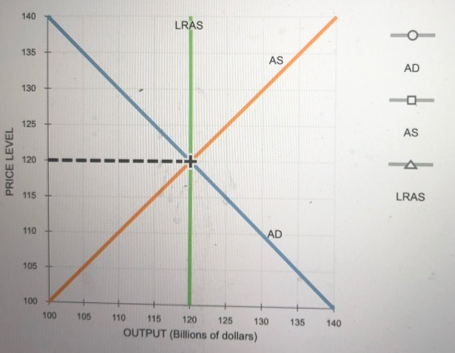9. Economic fluctuations II The following graph shows the short-run aggregate supply curve (AS), the aggregate demand curve (AD), and the long-run aggregate supply curve (LRAS) for a hypothetical economy. Initially, the expected price level is equal to the actual price level, and the economy is in long-run equilibrium at its natural level of output, \$120 billion. Suppose a bout of severe weather drives up agricultural costs, increases the costs of transporting goods and services, and increases the costs of producing goods...

• ### ()​-run equilibrium occurs at the intersection of the aggregate demand​ curve, ​AD, and the​ short-run aggregate...

()​-run equilibrium occurs at the intersection of the aggregate demand​ curve, ​AD, and the​ short-run aggregate supply​ curve, SRAS.() ▼ Long Short ​-run equilibrium occurs at the intersection of AD and the​ long-run aggregate supply​ curve, LRAS. Any unanticipated shifts in aggregate demand or supply are called aggregate demand or aggregate supply() ▼ shocks externalities . When aggregate demand decreases while aggregate supply is​ stable,() ▼ a recessionary an inflationary gap can​ occur, defined as the difference between how much...

• ### Unit 3: Aggregate Demand, Aggregate Supply, and Fiscal Policy AD, AS, and LRAS Short Run vs....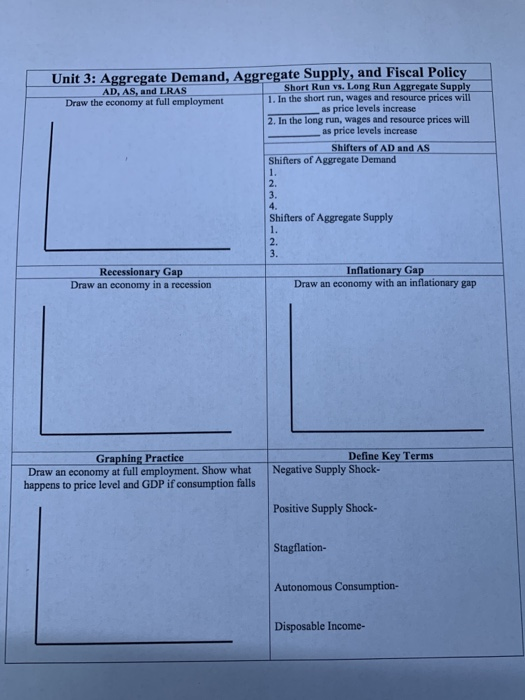Unit 3: Aggregate Demand, Aggregate Supply, and Fiscal Policy AD, AS, and LRAS Short Run vs. Long Run Aggregate Supply Draw the economy at full employment 1. In the short run, wages and resource prices will as price levels increase 2. In the long run, wages and resource prices will as price levels increase Shifters of AD and AS Shifters of Aggregate Demand Shifters of Aggregate Supply imi Recessionary Gap Draw an economy in a recession Inflationary Gap Draw an...

• ### What influences the LRAS (long run aggregate supply) and SRAS (short run aggregate supply)? What are...

What influences the LRAS (long run aggregate supply) and SRAS (short run aggregate supply)? What are the three theories that explain the upward slope of the SRAS? How do both monetary and fiscal policy affect the AD?

• ### The figure below depicts the aggregate demand curve (AD), the short-run aggregate supply curve (SRAS), and the long-run aggregate supply curve (LRAS) for the United States. The economy is initially at long-run equilibrium, at point A.The figure below depicts the aggregate demand curve (AD), the short-run aggregate supply curve (SRAS), and the long-run aggregate supply curve (LRAS) for the United States. The economy is initially at long-run equilibrium, at point A.One of the most contentious issues among economists involves the economy’s adjustment to long-run equilibrium. Some economists believe that adjustment can and should occur naturally. This group, the classical economists, stress the importance of aggregate supply. Others see the return to long-run equilibrium as an...